by Rachel Costa

## In the second part of my mini series about predictive analytics, I will show you the steps to perform regression analysis in Alteryx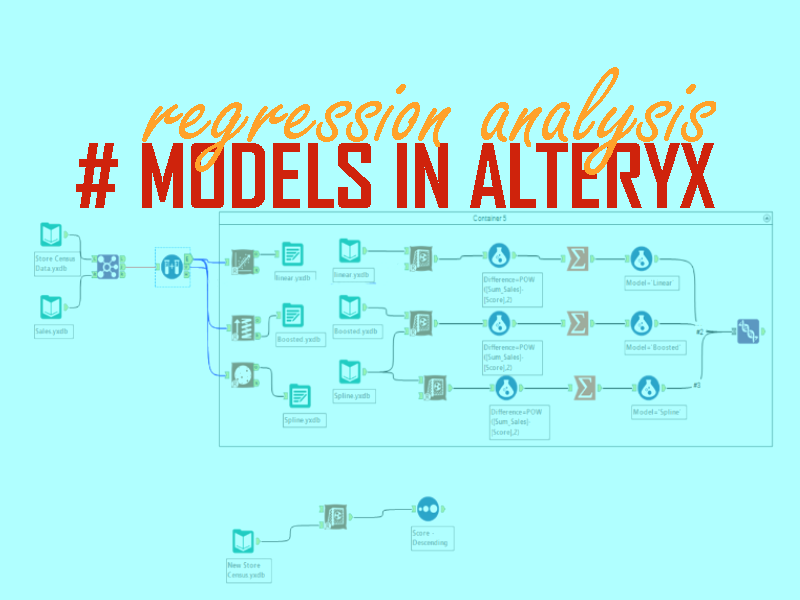As promised on my first post about Predictive Analytics (you can find it here), here is the second example I presented during the class. This time I will show how Alteryx can help you to carry out regression analysis.

Before going to the Alteryx workflow, a brief explanation: regression analysis is a type of “supervised learning” technique used in Predictive Analytics.

The name “supervised learning” explains the process: in this technique, we first test the algorithm to see if it works as we expect.

We can imagine this part as if we were formulating a question which we already know the answer and then asking it to the algorithm to check if its answer will be similar to the answer we already have – like the teacher does with his/her students to check if they have learned a topic taught in class.

Only after training the algorithm we apply it to the data we would like to create a prediction.

### Why to use regression analysis

Regressions analysis are a useful way to investigate the causal effect of one or more variables upon another. For instance, how energy consumption is affected by seasons? How to identify when a country is at high risk of an economic crisis?

There are lots of questions that can be predicted by regression analysis. In the example below we will discover how demographic data (as population background, income and other factors) impact on store sales. Then we will use these findings to predict which store a hypothetical company should open first from a list of options.

### REGRESSION ANALYSIS IN ALTERYX

We can split the regression analysis process in three main parts:

First: Training the algorithm with part of the data we have from real stores to see how the variables impact on sales (Working with the past = the dataset about real stores)

Second: Validating it using the remaining part of the real stores’ data

Third: Picking the best one to apply to the list of possible places to a new store, to predict which store would have the best total sales (Working with the future = the algorithm will apply what it learnt from the real stores to predict the performance of new stores).

The full workflow in Alteryx will look like the example bellow.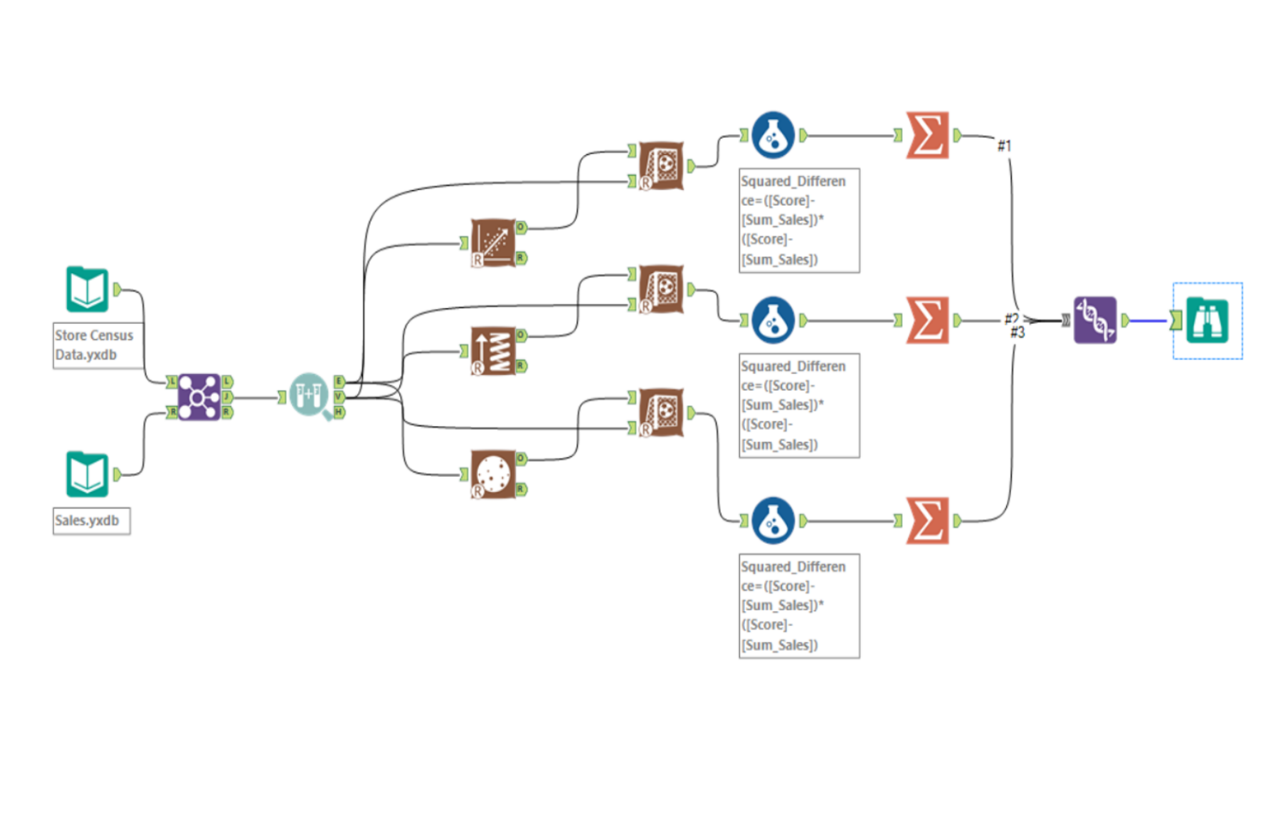### Training the Algorithm

The first thing we need to do when training the algorithm is to create samples of our data. Part of the data will be used to create an estimation and the remaining to validate the estimation.

Remember: the main objective in this first part is to compare the algorithm’s answer to the answer we already have. In other words, how near the real sales will be the algorithms prediction of those sales.

There is a tool in Alteryx called “Create Samples” that allows us to easily split our data in parts. You only need to drag and drop it to the workflow and configure it.

For this exercise we will use 80% of the data as the estimation sample and 20% as the validation sample (you can try it with other percentages, only paying attention to the fact that both, estimation and validation, when added up must be under 100%).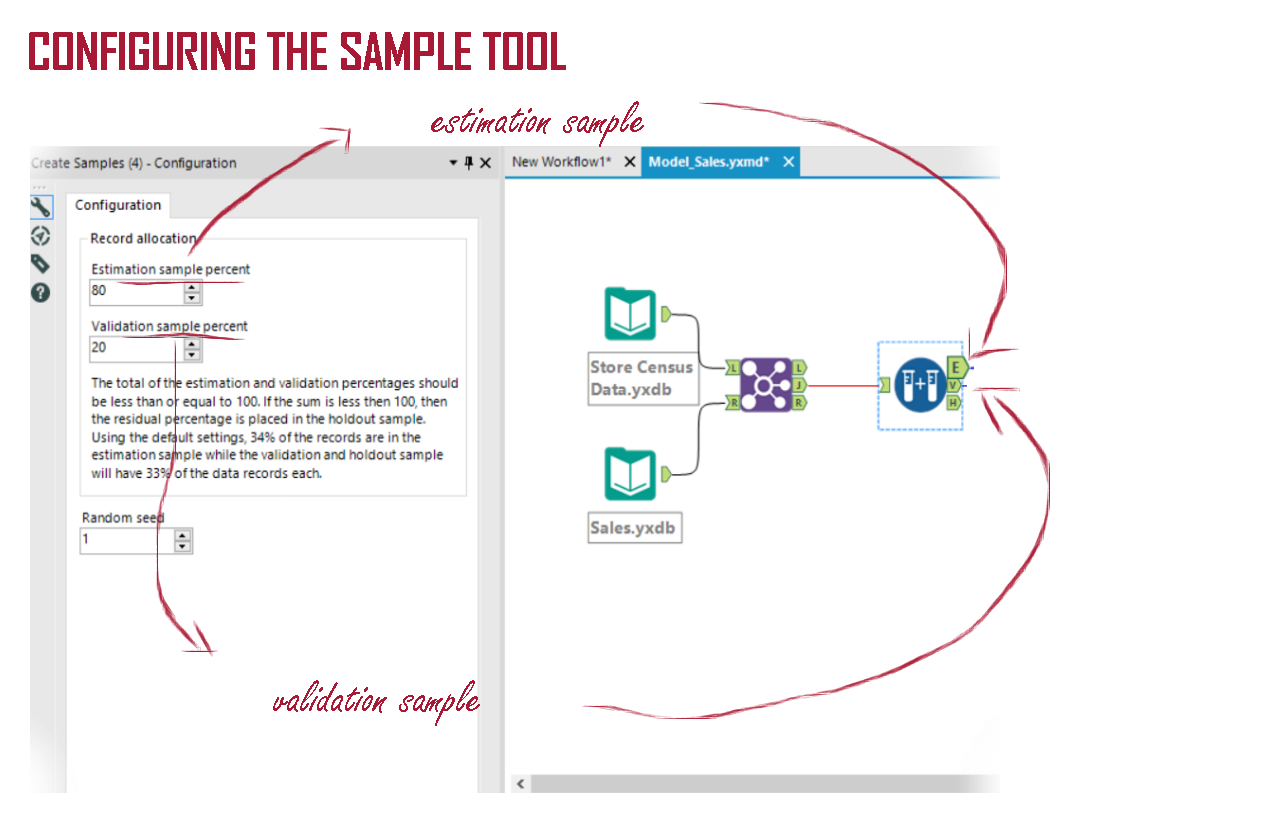Once we split the data, the next step is to compare different models to check which of them will give us the best answer. For this situation, as all our variables are quantitative (sales is quantitative as well as our predictors), we can use different types of regression model like the linear regression, the boosted model and the spline model (they are all good options to work with quantitative data).

In Alteryx, we will do this performing a series of tasks:

1. Connect the estimation sample to the different models. All the models mentioned above have a tool in Alteryx, all you need to do is to drag and drop the tools into the workflow.

1. Configure the models defining which is the target variable (the variable you would like to predict) and what are the predictor variables (the variables you think that can influence the target).

1. Run Alteryx.

1. Each one of the mentioned tools has an ‘O’ and a ‘R’. The ‘R’ is a report explaining how Alteryx did the calculation. The ‘O’ is the object, that we will apply to the validation sample.

However, to connect object and validation you will need something else: a tool called ‘Score’. It will add a column to the original data containing the predicted sales.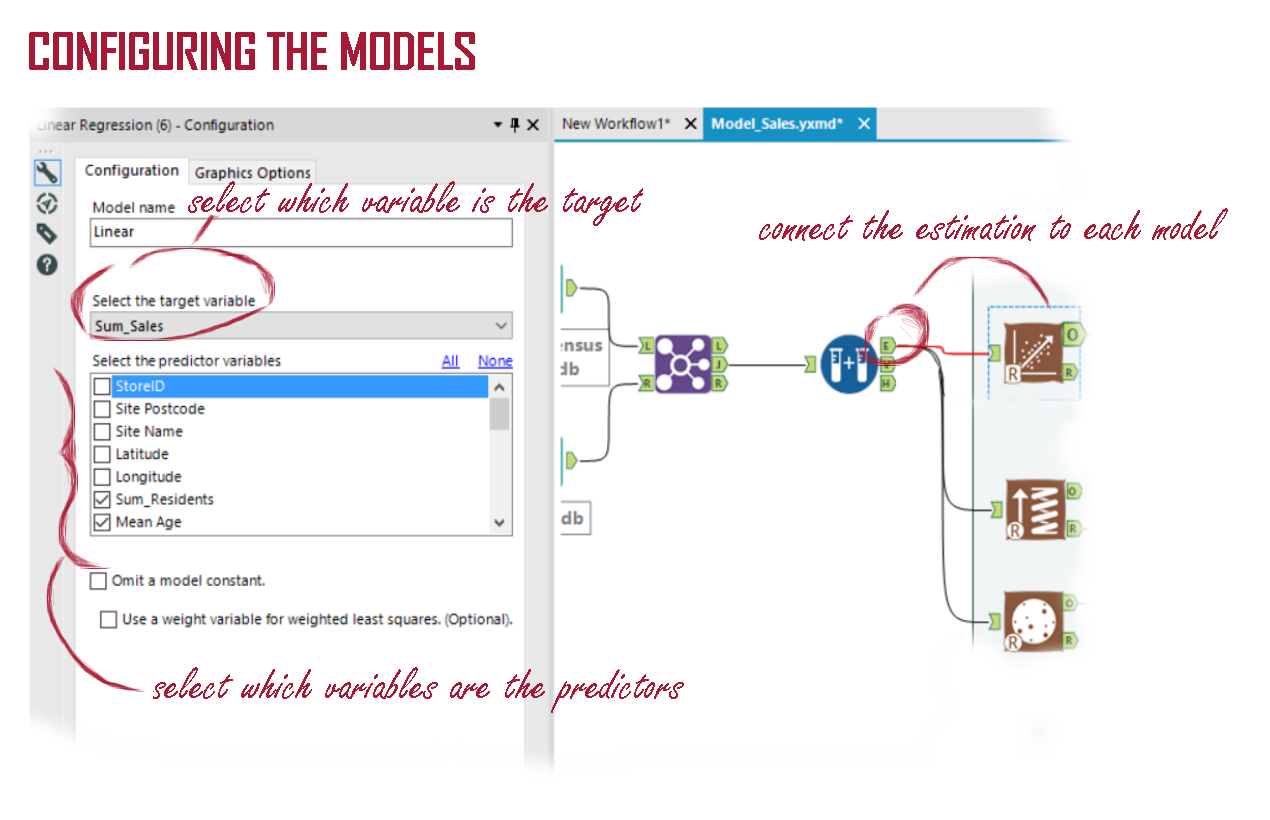### The validation process

Once you have the predicted sales value you can compare it to the real sales to discover which of the models predicted the nearest value.
The first step is to create a formula that will give you the difference between the real sales and the predicted sales for each row.

There is a trick thing in this part: the following step after the subtraction is to sum the difference for all rows and then compare the results – the smaller the number, the better the model.

For this reason, it is important to avoid negative values, once they could create the false impression that the model is better than it really is.

Why is it important? Imagine if the difference in one row is -8 and in another row is +8. When you add up those rows the result will be 0. It would give the impression that the predicted values have the same value than the real value, what is not true.

The solution to avoid this kind of misleading is to use the squared difference, once it will guarantee always positive results. In Alteryx, the function POW can be used to simplify the process. It returns the variable raised to the defined power.

In this case, the formula would be POW([Sum_Sales]-[Score],2).

Remember when configuring the tool formula to give a name to the new column you are creating and to define the type of variable you are creating (in this example, I am defining it as a “double”, that is one of the various ways to save numbers in Alteryx).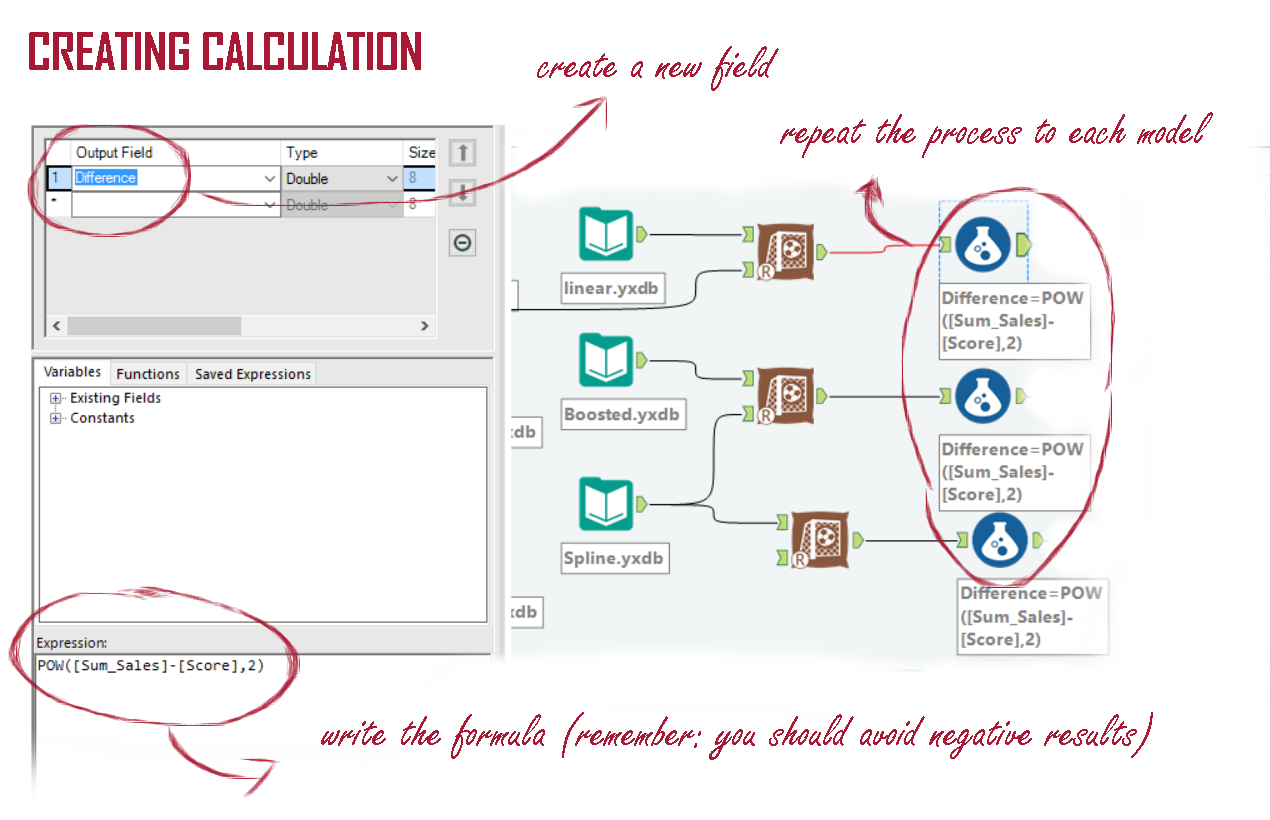With the new column added to your data, all you need to do is to add up the values of the new column using the “Summarise” tool.

To make the comparison easier, drag and drop a formula to each one of the models creating a new column in which you will store the name of the model.

Pay attention to give the same name for all columns, this way you can perform a union in the next step, creating a new data set containing two columns with three rows each.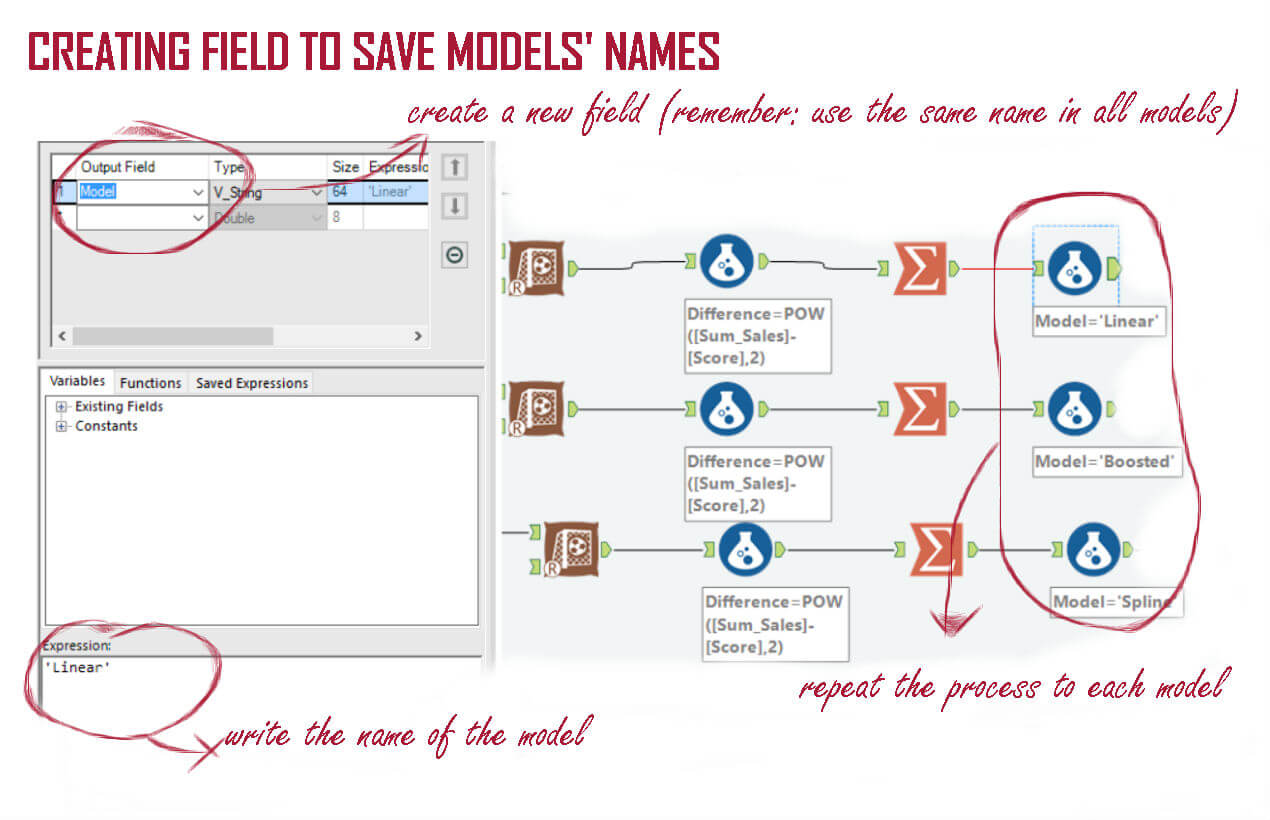Now all you need to do is to compare the rows to check which model predicted the nearest value to the real sales. In the case I presented it was the boosted model.

So, the next step is to (finally!) use this model to create a prediction to the new stores.

### Creating a prediction to the new stores

Now we have defined the best model, we can finally input the data from the new stores and start to work with them.

For this, we will use the “Score” tool again, this time connecting the data from the news stores and the object generated when testing the algorithm.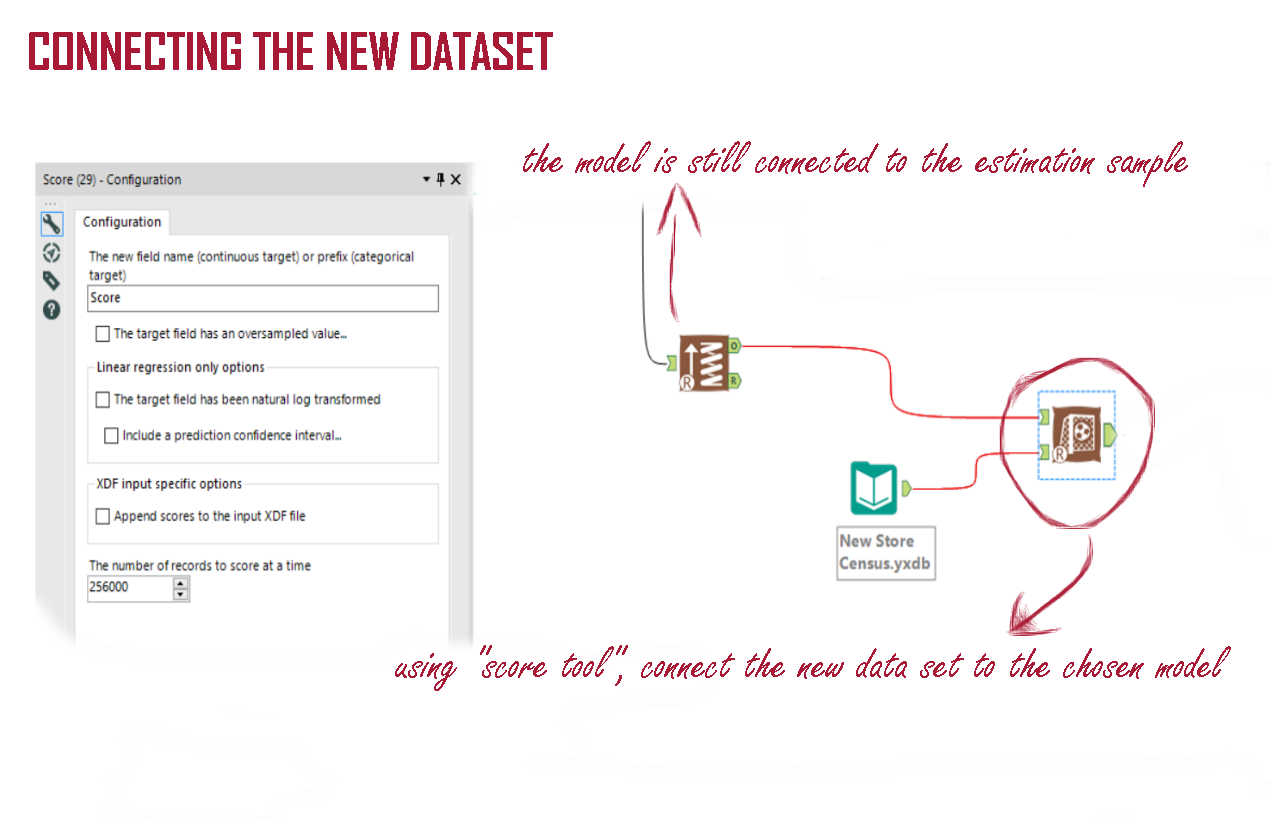Once again, it will generate a new column to your data with the predicted sales for each one of the non-existent stores.
The final step is to order the predicted sales to discover which one is the biggest. Drag and drop the “Sort” tool and order the new column descending.

Voilà! The best store to open is the “Store 8”!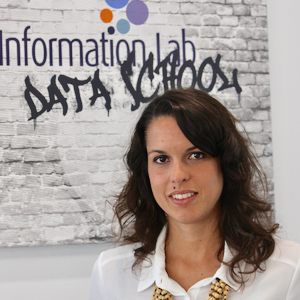Rachel Costa

Fri 30 Dec 2016

Fri 23 Dec 2016